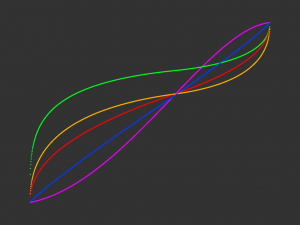# S-Curve Algorithm in ProcessingThe S-Curve algorithm I implemented in this example using Processing can be used for mapping values from a linear sequence to an s-curve.

GitHub repoA forum user wanted a parametric S-Curve passing through specified coordinates. I implemented several versions of the algorithm in the thread:

1. curve passes through point (a,a) (line with slope 1)
2. curve passes through (a,a) with endpoint slope influence
3. curve passes through (a,b) with endpoint slope influence. This is the most responsive curve with the mouse-point intersection requested in the thread.
4. simple curve through (0.5, 0.5) with endpoint slope influence
5. bezier curve with handle positioning proportional to (a,b) ratio

Keyboard Input

• left/right — decrease/increase inflection point slope (general strength of effect modifier)
• up/down — increase/decrease slope of endpoints
• h — show/hide chart features and text of slopes and cursor coordinates
• c — show/hide cursor
• s — save image